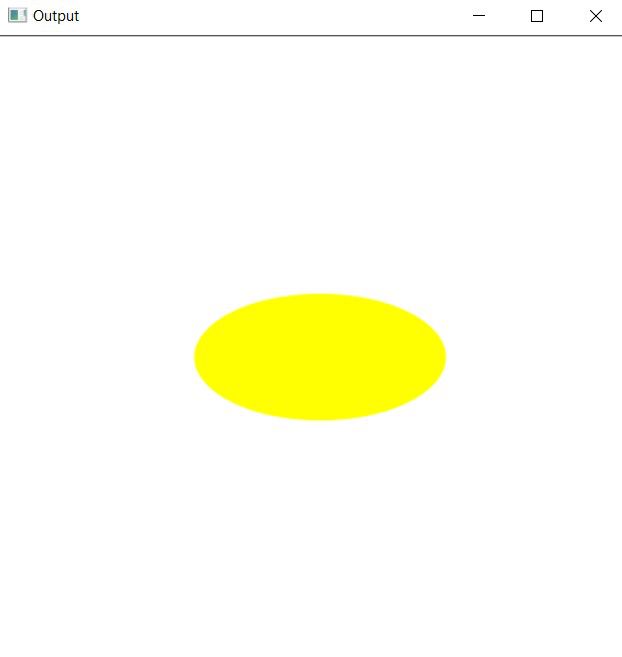# Draw an ellipse using OpenCV in C++

• Difficulty Level : Hard
• Last Updated : 22 Feb, 2021

In this article, the task is to draw an ellipse using OpenCV in C++. The ellipse() function from OpenCV C++ library will be used.

Syntax:

Want to learn from the best curated videos and practice problems, check out the C++ Foundation Course for Basic to Advanced C++ and C++ STL Course for foundation plus STL.  To complete your preparation from learning a language to DS Algo and many more,  please refer Complete Interview Preparation Course.

ellipse(image, centerCoordinates, axesLength, angle, startAngle, endAngle, color, thickness, lineType, shift)

Parameters:

• image: It is the image on which ellipse is to be drawn.
• centerCoordinates: Coordinates of the center of the ellipse. (Tuple of two coordinates (X-coordinate, Y-coordinate))
• axesLength:Tuple containing the major and minor axis of the ellipse (major axis length, minor axis length).
• angle: Ellipse rotation angle in degrees.
• startAngle: Starting angle of the elliptic arc(degrees).
• endAngle: Ending angle of the elliptic arc(degrees).
• color: It is the color of the borderline of the ellipse to be drawn. A tuple representing 3 colors (B, G, R) i.e., (Blue, Green, Red).
• thickness: It is the thickness of the ellipse borderline in px. The thickness of -1 px will fill the ellipse shape by the specified color.
• lineType: Type of the line. There are 3 types of line:
• LINE_4: Line was drawn using 4 connected Bresenham algorithm.
• LINE_8: Line was drawn using 8 connected Bresenham algorithm.
• LINE_AA: It draws Anti-aliased lines formed by using a Gaussian filter.
• shift: Number of fractional bits in the point coordinates.

Return Value: It returns an image.

Program 1:

Below is the C++ program demonstrating how to draw an ellipse over a self-formed background image:

## C++

 `// C++ program to demonstrating ellipse``// over a self-formed background image``#include ``#include `` ` `// Drawing shapes``#include `` ` `#include ``using` `namespace` `cv;``using` `namespace` `std;`` ` `// Driver Code``int` `main(``int` `argc, ``char``** argv)``{``    ``// Creating a blank image with``    ``// white background``    ``Mat image(500, 500, CV_8UC3,``              ``Scalar(255, 255, 255));`` ` `    ``// Check if the image is created``    ``// successfully or not``    ``if` `(!image.data) {``        ``std::cout << ``"Could not open or "``                  ``<< ``"find the image\n"``;`` ` `        ``return` `0;``    ``}`` ` `    ``// Drawing the ellipse``    ``ellipse(image, Point(256, 256),``            ``Size(100, 50), 0, 0,``            ``360, Scalar(0, 255, 255),``            ``-1, LINE_AA);`` ` `    ``// Showing image inside a window``    ``imshow(``"Output"``, image);``    ``waitKey(0);`` ` `    ``return` `0;``}`

Output:Explanation: In the above program, an ellipse is drawn at an angle of 0 degrees i.e., horizontal ellipse.

Program 2:

Below is the C++ program illustrate rectangle over a loaded image with an ellipse around the GFG logo:

## C++

 `// C++ program to demonstrate rectangle``// over a loaded image with an ellipse``// around the GFG logo``#include ``#include `` ` `// Drawing shapes``#include `` ` `#include ``using` `namespace` `cv;``using` `namespace` `std;`` ` `// Driver Code``int` `main(``int` `argc, ``char``** argv)``{``    ``// Reading the Image``    ``Mat image = imread(``"C:/Users/harsh/Downloads/geeks.png"``,``                       ``IMREAD_COLOR);`` ` `    ``// Check if the image is created``    ``// successfully or not``    ``if` `(!image.data) {``        ``std::cout << ``"Could not open or "``                  ``<< ``"find the image\n"``;``        ``return` `0;``    ``}`` ` `    ``// Drawing the ellipse``    ``ellipse(image, Point(115, 110),``            ``Size(105, 55), 0, 0,``            ``360, Scalar(0, 255, 255),``            ``1, LINE_AA);`` ` `    ``// Show our image inside a window``    ``imshow(``"Output"``, image);``    ``waitKey(0);`` ` `    ``return` `0;``}`

Output:My Personal Notes arrow_drop_up